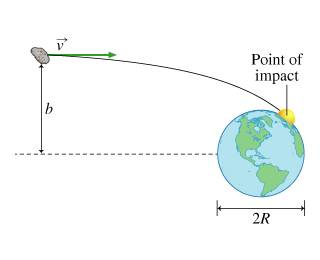# Cross Section for Asteroid Impact

• ebjessee

## Homework Statement

In this problem, you will estimate the cross section for an earth-asteroid collision. In all that follows, assume that the Earth is fixed in space and that the radius of the asteroid is much less than the radius R of the earth. The mass of the Earth is M_e, and the mass of the asteroid is m. Use G for the universal gravitational constant.E_initial = (m/2)v2)
L_initial = bmv
E_at surface of Earth = ((v_f)2*m)/2 +(GmMe)/R
L_at surface of Earth = (mvfR
bmax2 = R2+(2RGMe)/v2

Where I get confused is the actual significance of bmax:

The collision cross section S represents the effective target area "seen" by the asteroid and is found by multiplying (bmax)2 by π. If the asteroid comes into this area, it is guaranteed to collide with the earth.
A simple representation of the cross section is obtained when we write v in terms of ve, the escape speed from the surface of the earth. First, find an expression for ve, and let v=Cve, where C is a constant of proportionality. Then combine this with your result for (bmax)2 to write a simple-looking expression for S in terms of R and C.

Express the collision cross section in terms of R and C.

I know that a version of ve is sqrt(2GM/R), but I have no idea how to go on from here.

Did you take your expression for ve and form v = Cve? What did that do for your bmax2 equation? Show your work.

Ok. We say that v=Cve
So bmax2 = R2 + (2RGMe)/Cve2

The collision cross section S represents the effective target area "seen" by the asteroid and is found by multiplying (bmax)2 by π

Then π bmax2 = π R2 + 2π RGMe/Cve2

Distributing pi to both sides of the equations helps me see that I'm now looking at circular area equations, so that helps to but bmax into perspective. I plugged in ve = sqrt(2Gm/R) which helped me reduce the previous equation to:

S = π R2 +π R2Me/Cm

That didn't work. I got a message the answer did not depend on m or Me.

Nevermind- just forgot to square C and use the same Me. Thanks!

You want to replace ##v_e^2## in your ##b_{max}^2## equation with your expression for escape velocity right away and cancel out what can be cancelled. I don't know how m snuck into your equations...